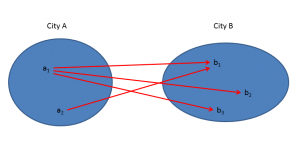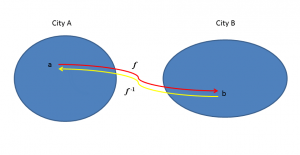30
Mar 18

## See if this definition of a function is better than others

Try the definitions from the Khan Academy, Math is Fun, WikipediaPaul's Online Math Notes, and there are some others. Most of them are obsessed with the input-output terminology, which is not used by professionals. I think my explanation is more visual.

## "Sending letters" is associated with arrows

Situation: every citizen$a$ from the city of$A$ sends letters to some citizen(s)$b$ of the city of$B$. In Figure 1, the arrows show that citizen$a_1$ sends letters to three citizens of$B$: they are$b_1,b_2,b_3$, while citizen$a_2$ sends letters to only$b_1$. Let us write$f(a)=b$ if$a$ sends letters to$b$. Here,$a$ is called an argument (some people say an input) and$b$ is called a value (some people say an output).Figure 1. This is not a function

Definition$f$ is called a function if for each argument, the corresponding value is unique.

The situation depicted in Figure 1 does not describe a function, because$a_1$ sends letters to more than one person. The visualization of a function is given in Figure 2, where arrows going from one citizen of$A$ to more that one citizen of$B$ are excluded.Figure 2. This is a function

We finalize the function definition with some remarks. In theory, of course, instead of cities, we talk about sets. Also, instead of "sending letters" we say "the function$f$ maps$a$ to$b$".$f(a)$ is called an image of$a$.

The set$A$ is called the domain of the function$f$. Go to the beginning of this section and see that we said "every citizen$a$ from the city of$A$..." This means that our function is defined everywhere in the domain. In practice, when you are given a function, you may have to describe the domain by removing all elements where your function is not defined.

## Here is a detective story

One citizen of$B$ mysteriously dies. Before dying, he manages to tell those around him that he had visited his correspondent in$A$ and was probably poisoned during the visit. The local detective's task will be simpler if the poor guy had been receiving letters from only one person. We write$f^{-1}(b)=a$ if$b$ receives letters from$a$. If nobody in$B$ receives letters from more than one sender, then$f^{-1}$ satisfies the definition of a function.

Definition. We say that the inverse function exists if$f^{-1}$ satisfies the definition of a function. Equivalently,

(1) whenever$a_1\ne a_2$, their images should be different:$f(a_1)\ne f(a_2)$.

In Figure 2, the inverse does not exist. In Figure 3, it does. The function$f$ takes us along the red arrow from$a$ to its image$f(a)=b$. The inverse function$f^{-1}$ takes us back along the yellow arrow from$b$ to$a=f^{-1}(b)$, which is called a counterimage (or preimage) of$b$.Figure 3. This function has an inverse

## How to find the derivative of the inverse

From now on the arguments and values will be real.

The direct function$f$ maps$a$ to$b$ and the inverse function maps$b$ back to$a$. This gives an obvious identity:$f^{-1}(f(a))=a$. Similarly,$f(f^{-1}(b))=b$. Since this is true for all$b$, we can differentiate this equation using the chain rule to get$f'(f^{-1}(b))\frac{df^{-1}(b)}{db}=1.$

Hence,$\frac{df^{-1}(b)}{db}=\frac{1}{f'(f^{-1}(b))},$ which is often written as$\frac{df^{-1}(b)}{db}=\frac{1}{f'(a)}$ with$a=f^{-1}(b).$

## Conditions sufficient for existence of the inverse

Condition 1. Let the function$f$ be strictly monotone. If it is increasing, for example, then this condition means that$x_1 implies$f(x_1). Obviously, this implies (1) and the inverse exists.

Condition 2 (condition sufficient for Condition 1). Suppose the derivative of$f$ does not change sign. For example, if the derivative is everywhere positive (think about the exponential function), then the function is strictly increasing and we can apply Condition 1.

Example. The function$f(x)=x^2$ is not one-to-one on the real line. If we take as its domain$[0,\infty)$, it will be one-to-one (it is strictly monotone and its derivative is positive on the set of positive numbers). On this set, its inverse is$f^{-1}(y)=\sqrt{y}$. The equations$f^{-1}(f(x))=x$ and$f(f^{-1}(y))=y$ take the form$\sqrt{x^2}=x$ and$(\sqrt{y})^2=y$. The equations$y=x^2$ and$x=\sqrt{y}$ are equivalent.